Math Olympiad Test: Triangles- 1

# Math Olympiad Test: Triangles- 1

Test Description

## 10 Questions MCQ Test Mathematics Olympiad for Class 9 | Math Olympiad Test: Triangles- 1

Math Olympiad Test: Triangles- 1 for Class 9 2022 is part of Mathematics Olympiad for Class 9 preparation. The Math Olympiad Test: Triangles- 1 questions and answers have been prepared according to the Class 9 exam syllabus.The Math Olympiad Test: Triangles- 1 MCQs are made for Class 9 2022 Exam. Find important definitions, questions, notes, meanings, examples, exercises, MCQs and online tests for Math Olympiad Test: Triangles- 1 below.
Solutions of Math Olympiad Test: Triangles- 1 questions in English are available as part of our Mathematics Olympiad for Class 9 for Class 9 & Math Olympiad Test: Triangles- 1 solutions in Hindi for Mathematics Olympiad for Class 9 course. Download more important topics, notes, lectures and mock test series for Class 9 Exam by signing up for free. Attempt Math Olympiad Test: Triangles- 1 | 10 questions in 10 minutes | Mock test for Class 9 preparation | Free important questions MCQ to study Mathematics Olympiad for Class 9 for Class 9 Exam | Download free PDF with solutions
 1 Crore+ students have signed up on EduRev. Have you?
Math Olympiad Test: Triangles- 1 - Question 1

### If one angle of the triangle is equal to the sum of the other two angles then the triangle is

Detailed Solution for Math Olympiad Test: Triangles- 1 - Question 1

Let the angles of triangle be x, y and
180° - ( x + y)
∴ 180° - (x + y) = (x + y)
⇒ 2(x + y) = 180°
⇒ x + y = 90°
∴ (180°- (x + y))  = 180° - 90° = 90°
∴ The triangle will be right angled triangle

Math Olympiad Test: Triangles- 1 - Question 2

### Find x in the given figure.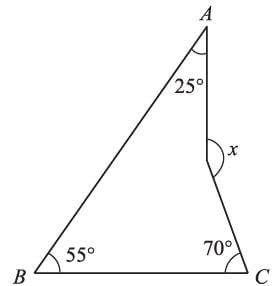Detailed Solution for Math Olympiad Test: Triangles- 1 - Question 2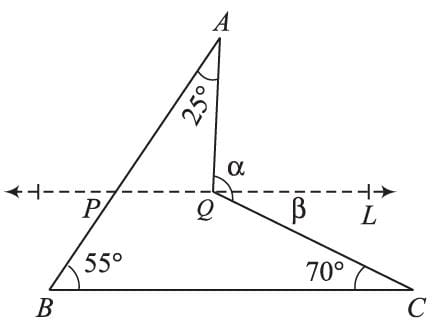Constructing a line PQ || BC,
∠APQ = ∠ABC = 55°
∵ ∠AQL is an exterior angle for ∠APQ
∴ ∠APQ + 25° = a
⇒ a = 25° + 55° = 80°
b = 70° (Alternate opposite ∠s)
∴ x = a + b = 70° + 80° = 150°

Math Olympiad Test: Triangles- 1 - Question 3

### If the bisectors of the acute angles of a right triangle meet at O, then the angle between the two bisectors at O is equal to:

Detailed Solution for Math Olympiad Test: Triangles- 1 - Question 3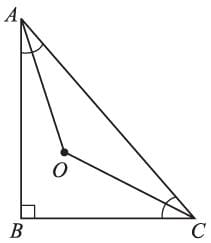∠AOC = 90º​​​​​​​ + 1/2∠​​​​​​​B
= 90º + 1/2 x 90º
= 90º +​​​​​​​ 45º
= 135º

Math Olympiad Test: Triangles- 1 - Question 4

Find x if BO and CO are the bisected of exterior angles at B and C respectively.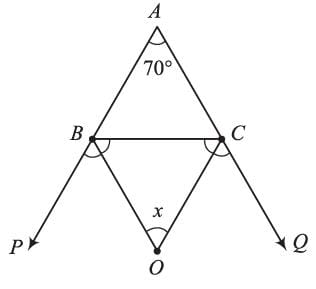Detailed Solution for Math Olympiad Test: Triangles- 1 - Question 4

x = 90° - 1/2∠A
= 90°​​​​​​​ - 1/2 x 70°
= 90°​​​​​​​ - 35°​​​​​​​ = 55°

Math Olympiad Test: Triangles- 1 - Question 5

The value of x from the adjoining figure will be: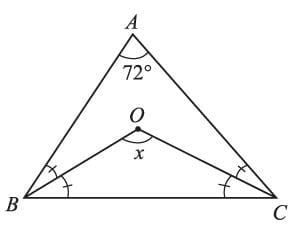Detailed Solution for Math Olympiad Test: Triangles- 1 - Question 5

x = 90° + 1/2∠A
= 90°​​​​​​​ + 1/2 x 72°
= 90°​​​​​​​ + 36°
= 126°

Math Olympiad Test: Triangles- 1 - Question 6

AB and CD are parallel lines and transversal EF intersects them at P and Q respectively. If ∠APR = 25°, ∠RQC = 30° and ∠CQF = 65° then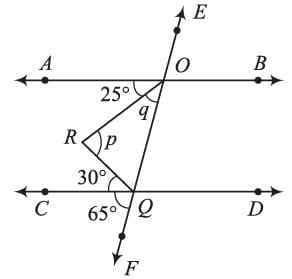Detailed Solution for Math Olympiad Test: Triangles- 1 - Question 6

∠APR + ∠CQF = 65°
(corresponding ∠s)
∠APR + ∠OPQ = 65°
⇒ 25° + Q = 65°
⇒ Q = 40°
∵ ∠OQF is on exterior angle for ∠POQ
∴ q + ∠POQ = 65° + 30°
⇒ p + q = 65°
⇒ p = 95° - 40° = 55°

Math Olympiad Test: Triangles- 1 - Question 7

The value of  x from the adjoining figure will be: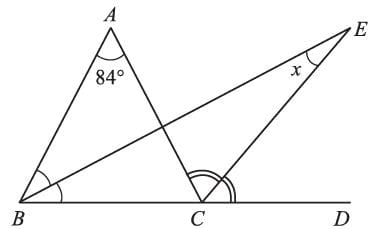Detailed Solution for Math Olympiad Test: Triangles- 1 - Question 7

∠ABC + ∠A = ∠ACD
⇒ ∠ACD = ∠ABC + 84°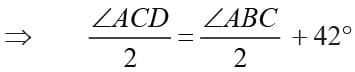⇒ ∠ECD = ∠EBC + 42° ...(i)
∵ ∠ECD is an exterior angle for ∠EBC
∴ ∠ECD =∠EBC = x ...(ii)
Comparing (i) and (ii), we get
x = 42°

Math Olympiad Test: Triangles- 1 - Question 8

ABC is a triangle in which ∠B = 2∠C. D is a point on BC such that AD bisects ∠BAC and AB = CD. BE is the bisector of ∠B. The measure of ∠BAC is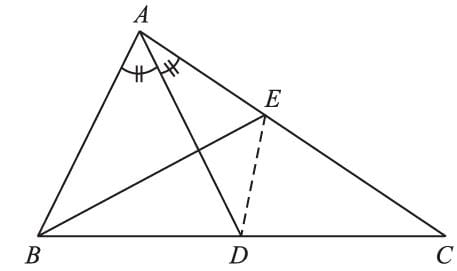Detailed Solution for Math Olympiad Test: Triangles- 1 - Question 8

In Δs ABC and DCE
∠ABE  = ∠DCE = ∠B/2
AB = CD (given)
BE = CE (∵ ∠ABE =∠DCE = ∠B/2)
∴ ΔABE ≅ ΔDCE [ By S-S-S congruence]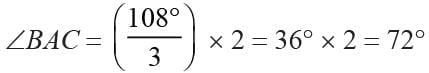Math Olympiad Test: Triangles- 1 - Question 9

ABCD is a quadrilateral having AC as a diagonal, then

Detailed Solution for Math Olympiad Test: Triangles- 1 - Question 9

In ΔABC
AB + BC > AC ...(i)
AD + DC > AC ...(ii)
Using (i) and (ii)
CD + AD + AB + BC > 2AC

Math Olympiad Test: Triangles- 1 - Question 10

In ΔABC, AC >AB and AD is the bisector of ∠A. Then

Detailed Solution for Math Olympiad Test: Triangles- 1 - Question 10

In ΔABC,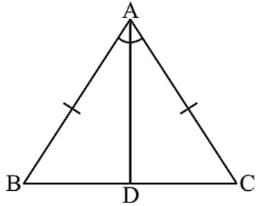∵ AC > AB
∴ ∠ABC = ∠ACB
⇒ - ∠ABC - ∠ACB
⇒ 180° - ∠ABC < 180° - ∠ACB
⇒ 180° - ∠ABC - x  < 180° - ∠ACB - x

## Mathematics Olympiad for Class 9

1 videos|43 tests
 Use Code STAYHOME200 and get INR 200 additional OFF Use Coupon Code
Information about Math Olympiad Test: Triangles- 1 Page
In this test you can find the Exam questions for Math Olympiad Test: Triangles- 1 solved & explained in the simplest way possible. Besides giving Questions and answers for Math Olympiad Test: Triangles- 1, EduRev gives you an ample number of Online tests for practice

## Mathematics Olympiad for Class 9

1 videos|43 tests